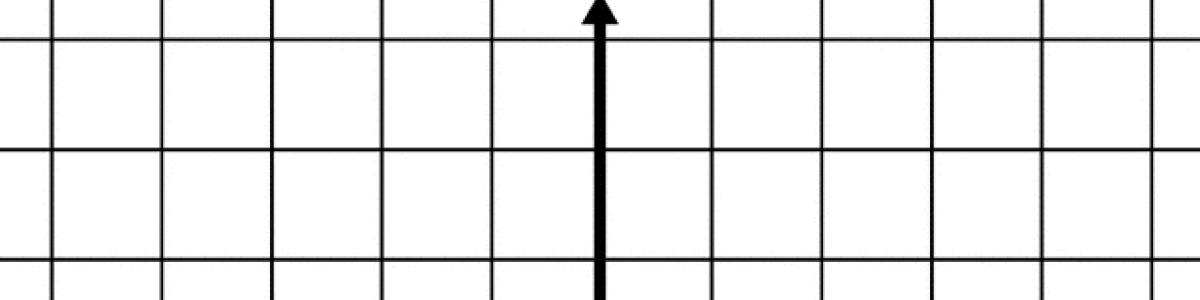Updated by rachelmx4 on May 18, 2018REPORT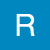rachelmx4
Owner
5 items   1 followers   0 votes   50 views

# Graphing Linear Equations Using Slope-Intercept Form

Targeted for high school algebra students, this list provides resources that will help students learn how to graph linear equations by using the slope-intercept form of the equation.

5

## Graphing Linear Equations - MathBootCamps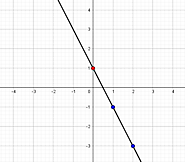This site demonstrates how to graph a line using three different methods. Method #2 describes how to use the slope and y-intercept from the slope-intercept form of an equation. It provides step-by-step instructions, images of graphs, and the slope formula.

2

## Algebra 1 – How to Graph a Linear Equation Using Slope and Y-Intercept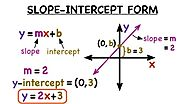Created by a foundation that is interested in the success of school-aged students, this site provides information for content in math and science. This particular link provides a simple 4 step method for graphing a line along with a color coded image to guide the student.

4

## Graphing Linear Equations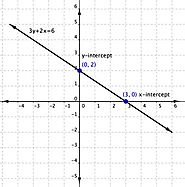For students who require a more detailed description of linear relationships, x- and y-intercepts, and using intercepts to graph lines, this site is the perfect resource. The site also provides topic terms and a glossary to promote a deeper understanding of the content.

1

## Graph from slope-intercept equation example | Algebra I | Khan Academy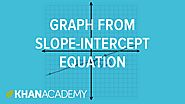Khan Academy provides a brief 3 minute video on the basics of graphing a line using slope-intercept form.

3

## Graphing a Linear Equation Using Slope Intercept Form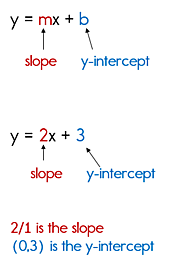This site provides step by step instructions, a video for clarification, and a brief list of rules to summarize the process.